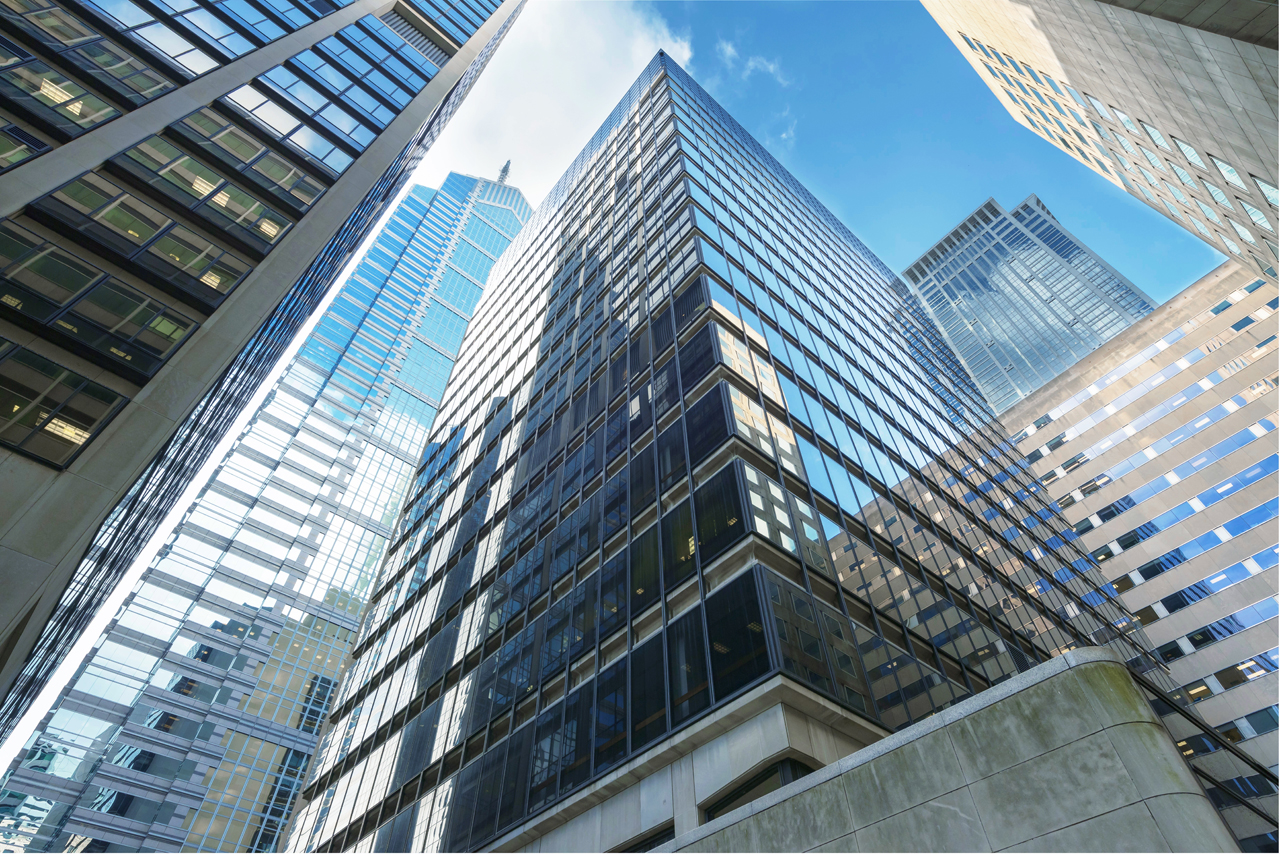If you aren’t immersed in the world of Commercial Real Estate Investing, a lot of the multifamily terminology may not be familiar to you. To make it easier, we have gathered a few of the most important terms to know. These terms are frequently used in relation to the purchase and financing of real estate assets. We are sourcing our definitions from NAIOP, which is a leading organization for developers, owners and investors of commercial real estate.

Understanding these terms will help you to make informed investment decisions. Every real estate investment is unique which increases the importance of being able to analyze and compare them using the metrics defined below.

## Important Commercial Real Estate Investing Terms:

##### Net Operating Income (NOI):

The income generated after deducting operating expenses but before deducting taxes and financing expenses.

NOI = (Rental Income + Other Income – Vacancy and Credit Losses) – Operating Expenses

NOI does not include loan payments, depreciation, amortization, or capital expenditures, and is always calculated before tax.

##### Debt Service:

The cash that is required to cover the repayment of interest and principal on a debt placed on the asset for a particular period.

##### Capital Expenses or Cap Ex:

Improvements (as opposed to repairs) to a fixed asset that will increase the value or useful life of that asset. A capital expenditure is typically amortized or depreciated over the useful life of the asset, as opposed to a repair, which is expensed in the year incurred.

##### Net Cash Flow:

Net cash flow is the annual income produced by an investment property after deducting allowances for debt service, capital expenditures, and other non-operating expenses from net operating income.

Net Income = NOI – (Debt Service + Capital Expenses + Other Non-Operating Expenses)

##### Cash on Cash Return (CoC):

CoC is used to measure the ratio between an asset’s annual cash flow in relation to the amount of capital invested. Like NOI CoC is also typically calculated before tax.

Cash on Cash Return = Annual Pre-Tax Cash Flow / Total Cash Invested

##### Return on Investment (ROI):

A measure of the value created by a real estate investment. It is the difference between the net gains from investing in the property less the net cost from investing in the property divided by the purchase price of the property. Usually, it is reported as a percentage.

ROI = (Current Asset Value – Cost of Investment) / Cost of Investment

Let’s say you purchased a property for \$800,000 and you put \$300,000 into renovations and you sell the property 3 years later for \$1,500,000.  The cost of the investment is \$800,000 + \$300,000 = \$1,100,000 ROI = (\$1,500,000 – \$1,100,000) / \$1,100,000 = .3636 or 36.36%.

##### Internal Rate of Return (IRR):

For income properties, it is the interest or discount rate needed to discount the sum of future net cash flows, including amortization and payments of loans and depreciation of the real property, to an amount equal to the initial equity of the property.

More simply stated, IRR is the discount rate at which the net present value of a set of cash flows (i.e., the initial investment, expressed negatively, and the returns, expressed positively) equals zero.

The IRR is useful because it can provide an “apples-to-apples” comparison of two cash flows with different distribution timing.

Example 1: If you invested \$100,000 and received a 10% annual distribution each year for 3 years and sell the asset for \$100,000 (the same amount you purchased it for), your IRR = 10%.

Example 2: If you invested \$100,000 and received no cash flow for 3 years and sold it at the end of year 3 for \$133,000, your IRR = 10%.

Notice that it takes more cash to achieve the same IRR in the second example (\$33,100 vs \$30,000).  This is because of the time value of money.

##### Capitalization Rate or Cap Rate:

Unlevered initial return from the acquisition of a real estate asset calculated by dividing net operating income (NOI) by the property sales price.

Cap Rate = Net Operating Income / Asset Market Value

For example, a property’s capitalization rate (cap rate) is 10 percent if it is purchased for \$10 million and produces \$1 million in NOI for one year. The cap rate is typically calculated using the NOI generated in the first year of ownership so investors can normalize and compare potential returns among competing investment properties. Also important is the Stabilized Cap Rate which is the ratio between the net operating income produced by a property upon achieving target occupancy and its purchase value.

##### Debt Coverage Ratio:

The debt coverage ratio is the ratio of the net operating income to the mortgage payment. If net operating income is projected to change over time, the investor typically reports the first year’s net operating income.

DCR = Net Operating Income / Total Debt Service

##### Loan to Value Ratio (LTV):

The ratio between a mortgage loan and the value of the property pledged as security, usually expressed as a percentage.

Loan to Value Ratio = Mortgage Amount / Appraised Asset Value

## Commercial Real Estate Investing with CEP

Do you have additional questions about these terms and want to learn more about what they mean to CEP? Reach out to us to set up a consultation.